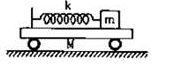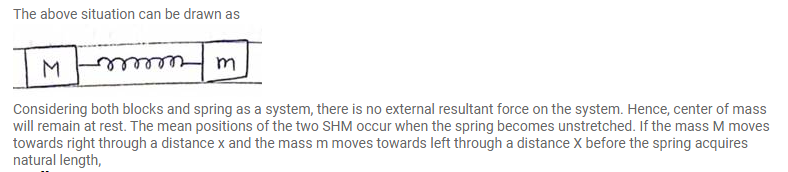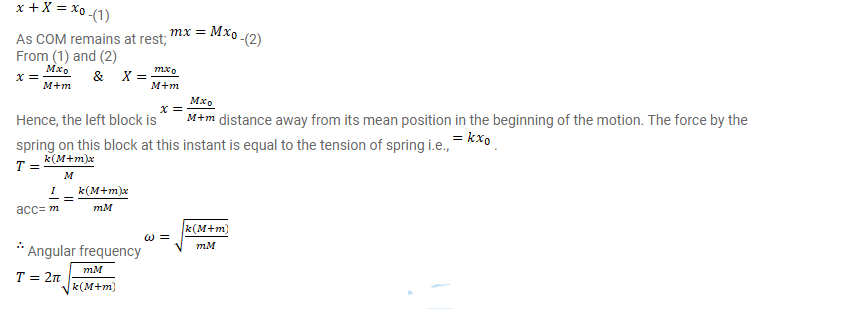# All the surfaces shown in figure are frictionless.

Question:

All the surfaces shown in figure are frictionless. The mass of the car is $\mathrm{M}$, that of the block is $\mathrm{m}$ and the spring has spring constant $k$. Initially, the car and the block are at rest and the spring is stretched through a length $x_{0}$ when the system is released.

(a) Find the amplitudes of the simple harmonic motion of the block and of the car as seen from the road.

(b) Find the time period(s) of the two simple harmonic motions.Solution: